Select Page

# Maths 12 Science CBSE Vector Algebra Answers for MCQ in English

Maths 12 Science CBSE Vector Algebra Answers for MCQ in English to enable students to get Answers in a narrative video format for the specific question.

Expert Teacher provides Maths 12 Science CBSE Vector Algebra Answers for MCQ through Video Answers in English language. This video solution will be useful for students to understand how to write an answer in exam in order to score more marks. This teacher uses a narrative style for a question from Vector Algebra not only to explain the proper method of answering question, but deriving right answer too.

Please find the question below and view the Answer in a narrative video format.

Question:

## Similar Questions from CBSE, 12th Science, Maths, Vector Algebra

Question 1 : Find the magnitude of two vectors a and b having the same magnitude and such that the angle between them isand their scalar product is 1/2. (View Answer Video)

Question 2 : Find a unit vector parallel to the sum of vectorsand(View Answer Video)

Question 3 : Find a vector in the direction of vectorwhich has magnitude 8 unit. (View Answer Video)

Question 4 : Find |x|, if for a unit vector a,(x - a).(x + a) = 12. (View Answer Video)

Question 5 :  Find the position vector of c which divides the line segment joining A & B whose position vectors areandinternally in the ratio

### Probability

Question 1 : Ifandfindif E and F are independent events.  (View Answer Video)

Question 2 : A bag I contains 5 red and 4 white balls and a bag II contains 3 red and 3 white balls. Two balls are transferred from the bag I to the bag II and then one ball is drawn from bag II. If the ball drawn from the bag II is red, then find the probability that one red ball and one white ball are transferred from the bag I to the bag II.   (View Answer Video)

Question 3 :  A and B throw a pair of dice alternatively, till one of them gets a total of 10 and wins the game. Find their respective probabilities of winning, if A starts first.   (View Answer Video)

Question 4 : Evaluate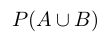, if 2P(A)=P(B)=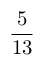and=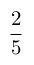. (View Answer Video)

Question 5 : A speaks truth in 75% of the cases, while B in 90% of the cases. In what percent of cases are they likely to contradict each other in stating the same fact? Do you think that statement of B is always true?     (View Answer Video)

### Determinant

Question 1 : The solution of the following system of equation is 2x + 3y = 5, 5x – 2y = 3. (View Answer Video)

Question 2 : Evaluate the determinant:. (View Answer Video)

Question 5 : Evaluate. (View Answer Video)

### Matrices

Question 1 : Compute:. (View Answer Video)

Question 2 : LetFind A + B. (View Answer Video)

Question 3 : If, write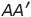, where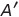is the transpose of A? (View Answer Video)

Question 4 : Find the value of z,  from the equation:(View Answer Video)

Question 5 : Find the inverse of the matrix,. (View Answer Video)